# Costas array

(diff) ← Older revision | Latest revision (diff) | Newer revision → (diff)

of orderAnpermutation matrix in which the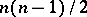line segments connecting pairs of ones in the matrix are distinct as vectors, i.e., no two agree in both magnitude and slope. Thus, if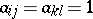andin the matrix, with,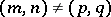, and at least three of these four ordered pairs are distinct, and if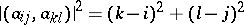equals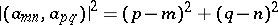, then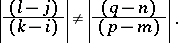All known (1996) general constructions for Costas arrays (see [a3], [a4], [a5]) involve primitive roots in finite fields (cf. Field). The Welch construction, for every prime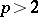, gives a Costas array of order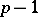by setting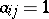when, whereis a primitive root modulo. Removing rowand columnfrom this construction leaves a Costas array of order. When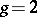is used as the primitive root modulo, the array can be further reduced to order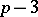.

In Golomb's construction, ifandare any two primitive elements in, for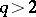, a Costas array of order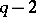is obtained by settingwhenever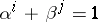. (The case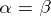had been discovered by A. Lempel.) If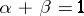, then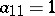, so that by removing the first row and first column, a Costas array or order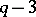is obtained. As shown in [a6], for everyone can find primitive rootsand(not necessarily distinct) with.

For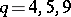, or any prime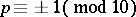, and for no other finite fields, there is a primitive rootwith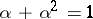. Thus, in the Lempel construction,, so that if the first two rows and first two columns are removed, a (symmetric) Costas array or orderresults. For, or any prime, there are primitive rootsandwithand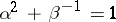. In this case,,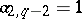and. By successive removal of rows and columns Costas arrays of orders,,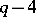, and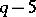are obtained for these values of.

In some cases a Costas array of ordercan be obtained from one of orderby adjoining ain an exterior corner, i.e., in one of the positions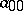,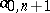,, or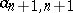. Several prime-order examples were obtained in this way from a Welch example of order.

The total numberof Costas arrays of orderis known (1996) for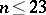(see the table), with a local maximum at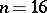.'

<tbody> </tbody>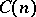2 2 13 12828 3 4 14 17252 4 12 15 19612 5 40 16 21104 6 116 17 18276 7 200 18 15096 8 444 19 10240 9 760 20 6464 10 2160 21 3536 11 4368 22 2052 12 7852 23 872

For a prime numberthere are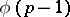primitive roots, each of which leads to a different Costas array of order. Sincecan be arbitrarily large,. It has been conjectured, but not proved, that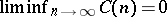. This would requireinfinitely often. No case of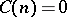is yet known, but no examples of Costas arrays of orders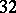,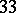, orhave yet been found. (Many larger orders also lack examples.)

J.P. Costas [a1] first proposed these arrays for an application to frequency hopping sonar signals. Let therows representequally spaced frequencies, and thecolumnsequal duration time intervals. Then the Costas array specifies a permuted order for thefrequencies to be transmitted inconsecutive time intervals. As a sonar (or radar) signal this design has an ideal "thumb-tack" ambiguity function (the two-dimensional auto-correlation function in time and frequency). The horizontal (time) shift measures range (the distance to the target) and the vertical (frequency) shift measures the Doppler (the velocity of the target relative to the observer). For any non-zero shift parallel to the coordinate axes a Costas array has at most one "hit" (coincidence of awith a), and thus gives the least ambiguous reading of the correct range and Doppler in the presence of noise.

 [a1] J.P. Costas, "Project medior. A medium-oriented approach to sonar signal processing" , HMED Tech. Publ. R66EMH12 , General Electric (1966) [a2] S.W. Golomb, H. Taylor, "Two-dimensional synchronization patterns with minimum ambiguity" IEEE Trans. Inform. Theory , 28 (1982) pp. 600–604 [a3] S.W. Golomb, H. Taylor, "Constructions and properties of Costas arrays" Proc. IEEE , 72 (1984) pp. 1143–1163 [a4] S.W. Golomb, "Algebraic constructions for Costas arrays" J. Combin. Th. A , 37 (1984) pp. 13–21 [a5] S.W. Golomb, "The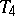andconstructions for Costas arrays" IEEE Trans. Inform. Th. , 38 (1992) pp. 1404–1406 [a6] O. Moreno, J. Sotero, "Computational approach to conjecture A of Golomb" Congressus Numerantium , 70 (1990) pp. 7–16 [a7] J.P. Costas, "A study of a class of detection waveforms having nearly ideal range-doppler ambiguity properties" Proc. IEEE , 72 (1984) pp. 996–1009
How to Cite This Entry:
Costas array. Encyclopedia of Mathematics. URL: http://encyclopediaofmath.org/index.php?title=Costas_array&oldid=11925
This article was adapted from an original article by S.W. Golomb (originator), which appeared in Encyclopedia of Mathematics - ISBN 1402006098. See original article